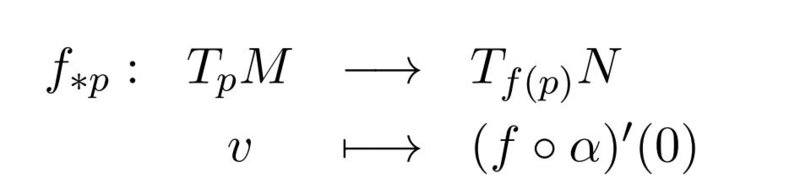# Differential of a function

• A
• Maxi1995
In summary, the conversation discusses the definition of the differential of a function on a submanifold, using the chain rule and the concept of pushforwards. It also provides an example of how this can be applied to a specific problem. The conversation concludes by confirming that the differential can be interpreted as the composition of functions with the chain rule.

#### Maxi1995

We define the differential of a function f in

$$p \in M$$,

where M is a submanifold as followsIn this case we have a smooth curve ans and interval I $$\alpha: I \rightarrow M;\\ \alpha(0)= p \wedge \alpha'(0)=v$$.

How can I get that derivative at the end by using the definitions of the derivative of a function in several variables?

#### Attachments

For an example, you can look at Problem No. 4 here:
Thank you for your answer. So I got it right, that it is possible to interpret the differential via the chain rule as $$(f \circ\alpha)'(0)=df(\alpha(0))*\alpha'(0)=df(p)v?$$
\begin{equation*} \begin{aligned} M &\;\quad \stackrel{f}{\longrightarrow} &N\\ \downarrow{\varphi}&&\downarrow{\psi}\\ \mathbb{R}^m &\;\quad \stackrel{g}{\longrightarrow} &\mathbb{R}^n \end{aligned} \end{equation*}